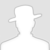FreeBuf.COM网络安全行业门户，每日发布专业的安全资讯、技术剖析。FreeBuf+小程序

CTF 图像隐写Python脚本处理
2019-06-24 15:02:46

CTF中经常会遇到很多图片的隐写题目需要使用脚本来解题，最常用到的就是使用python中的PIL库，所以如果要更好的解出图片隐写相关处理的题目，掌握好这个库的使用是必要的。本期就来给大家介绍下这个库的基本使用和几道图片题目的解题思路。

## 0x00 PIL vs Pillow

```pip install Pillow
```

## 0x01 Image 类

```from PIL import Image
```

### 1.加载图片，使用方法open

```from PIL import Image
im = Image.open('Mycat.jpg')
```

im是一个Image对象，属性有format，size，mode。format是格式，size 是一个元组，表示`(宽，高)`，mode则指的图片的模式。

```from PIL import Image
im = Image.open('Mycat.jpg')
print im.format,im.size,im.mode
```

console输出如下：

```JPEG (245, 280) RGB
```

### 2.呈现图片，方法show方便用来调试和测试。

```from PIL import Image
im = Image.open('Mycat.jpg')
im.show()
```

### 3.图片的读和写

```infile = 'Mycat.jpg'
f,e = os.path.splitext(infile)
outfile = f + '.png'
try:
Image.open(infile).save(outfile)
except IOError:
print "cannot convert",infile
```

### 4.图片的剪切，黏贴

1. 图片剪切

```im = Image.open('Mycat.jpg')
box = (150,150,245,280)
region = im.crop(box)
region.show()
```2. 图片黏贴

```im = Image.open('Mycat.jpg')
box = (50,50,200,200)
region = im.crop(box)
# 将图片逆序旋转180后，黏贴到原来复制的位置
region = region.transpose(Image.ROTATE_180)
im.paste(region,box)
im.show()
```

### 5.图像序列

```from PIL import Image
im = Image.open("laopo.gif")
im.seek(1)
im.show()

try:
while 1:
im.seek(im.tell()+1)
im.show()
except EOFError:
pass
```

```from PIL import Image
from PIL import ImageSequence

im = Image.open("laopo.gif")
for frame in ImageSequence.Iterator(im):
frame.show()
```

### 6.读取像素和修改像素

```from PIL import Image
img = Image.open('Mycat.jpg')
width , height = img.size
for i in range(0,width):
for j in range(0,height):
tmp = img.getpixel((i,j))
img.putpixel((i,j),(0,0,tmp))
img.show()
```

## 0x02 CTF 图片隐写实战

### 题目1：图片内容读取```# coding:utf-8
# author:Reborn

f1 = open('flag.jpg','rb+')
f2 = open('fla.jpg','wb+')
f1.close()
f2.close()
```### 题目2：GIF图片分离并合并```# coding:utf-8
# author:Reborn
from PIL import Image

savepath = "OmyGods\\"
path = '.\\'

im = Image.open('glance.gif')
try:
im.save(savepath+'glance{:d}.png'.format(im.tell()))
while True:
im.seek(im.tell()+1)
im.save(savepath+'glance{:d}.png'.format(im.tell()))
except:
pass
```

```# coding:utf-8
# author:Reborn
from PIL import Image
path = "OmyGods\\"
save_path = '.\\'

im = Image.new('RGBA',(2*201,600))

imagefile = []
width = 0
for i in range(201):
imagefile.append(Image.open(path+'Frame'+str(i)+'.png'))

for image in imagefile:
im.paste(image,(width,0,2+width,600))
width = width +2
im.save(save_path+'OmyGod.png')
im.show()
```

### 题目3：判断图片颜色```0.jpg:(白色)
(46656, (255, 255, 255))
1.jpg:(黑色)
(46656, (12, 12, 0))
2.jpg:(黑色)
(46656, (12, 12, 0))
3.Jpg:(白色)
(46656, (255, 255, 255))
......
```

```# coding:utf-8
# author:Reborn
from PIL import Image
path = "gif\\"
save_path = '.\\'

sumDo = '0b'
sumNo = '0b'
imagefile=[]
for i in range(104):
imagefile.append(Image.open(path+str(i)+'.jpg'))
for image in imagefile:
if image.getcolors() == 12:
sumDo +='1'
sumNo +='0'
else:
sumDo +='0'
sumNo +='1'

print (hex(eval(sumDo))[2:-1]).decode('hex')
print (hex(eval(sumNo))[2:-1]).decode('hex')
```

### 题目4：LSB处理

```# coding:utf-8
# author : reborn

from PIL import Image
img = Image.open('01.bmp')
width,height=img.size
print img.mode
for i in range(0,width):
for j in range(0,height):
tmp = img.getpixel((i,j))
print '==============='
print "原本像素为：",tmp
print bin(tmp)
print "000000001 与运算结果："
print tmp&0x1
``````# coding:utf-8
# author : reborn

from PIL import Image
img = Image.open('01.bmp')
width,height=img.size
for i in range(0,width):
for j in range(0,height):
tmp = img.getpixel((i,j))
if tmp&0x1 == 0:
img.putpixel((i,j),0)
else:
img.putpixel((i,j),255)
img.show()
```最终获得一张二维码，扫描该二维码可得flag。

## 0x03 最后# python # CTF 图像隐写

+ 收入我的专辑• 0 文章数
• 0 评论数
• 0 关注者